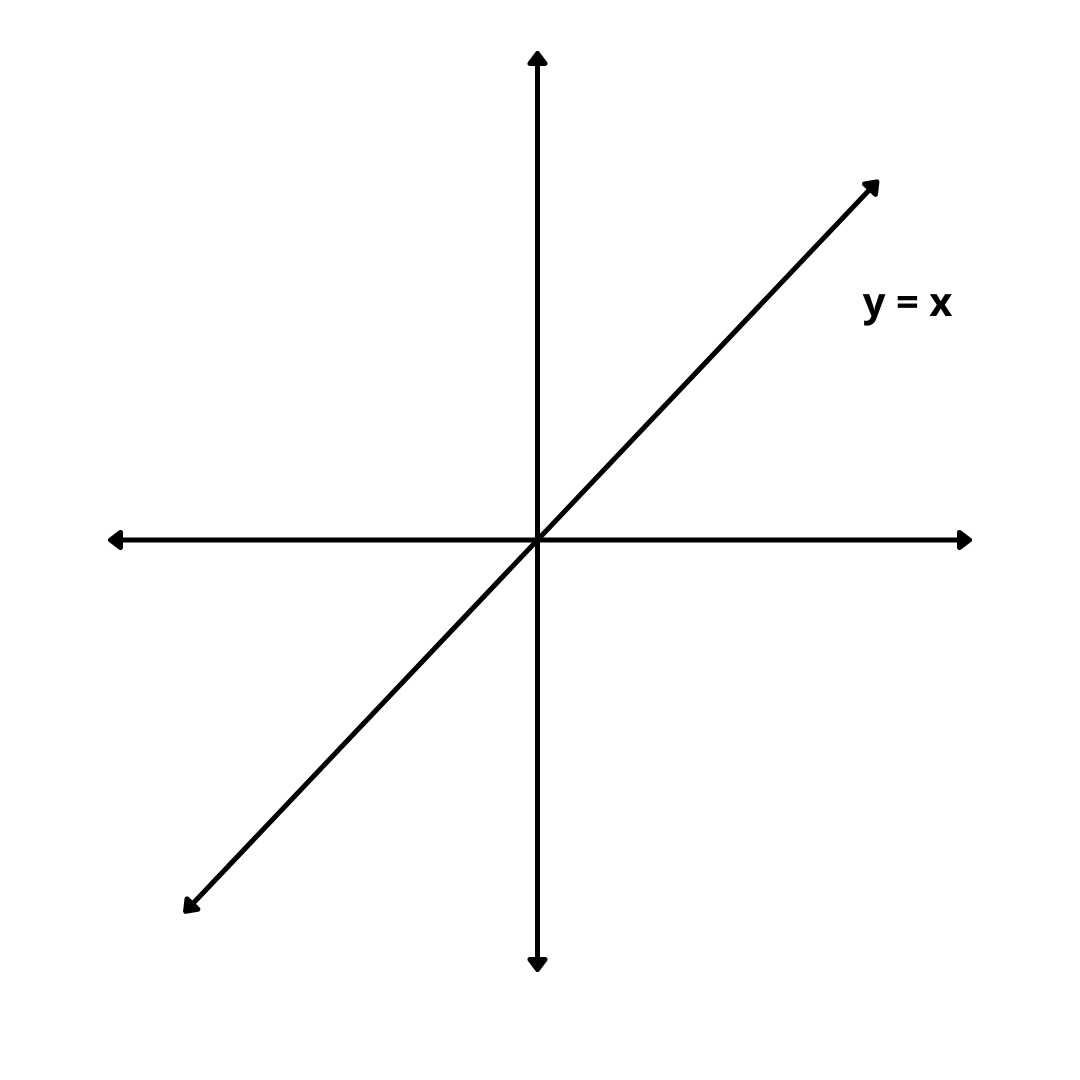# Identity Function – Definition, Graph, Domain and Range

Here you will learn what is identity function with definition, graph and examples.

Let’s begin –

## What is Identity Function ?

Definition : The function that associates each real number to itself is called the identity function. It is usually denoted by I.

Thus, the function I : R $$\rightarrow$$ defined by I(x) = x for all x $$\in$$ R is called the identity function.

For Example, f(2) = 2

## Graph of Identity Function

The graph of a function f(x) = x or y = x is a straight line passing through the origin and inclined at an angle of 45 degrees with x-axis.## Domain and Range

Clearly, the domain and range of the identity function are both equal to R.

Domain : R

Range : R